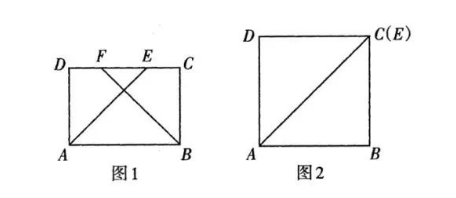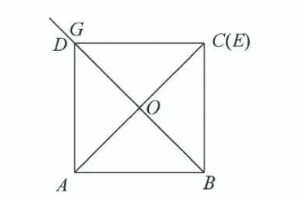(1) 如图 1, $\angle A B C$ 的角平分线 $B F$ 交边 $C D$ 于点 $F$, 求证: $D F=C E$;
(2) 如图 2, 当点 $E$ 与点 $C$ 重合时.
(1) 作 $\angle A B C$ 的角平分线 $B G$ 交 $A C$ 于点 $O$;
(2)当 $A B=4$ 时,求(1)中 $B O$ 的长.【答案】 解: (1) $\because$ 四边形 $A B C D$ 是矩形, $\therefore A D=B C, D C / / A B$.
\begin{aligned} & \therefore \angle E A B=\angle D E A, \angle A B F=\angle B F C . \\ & \because \angle D A B, \angle A B C \text { 的平分线 } A E, B F \text { 与边 } C D \text { 分别交于点 } E \text { 和 } F, \\ & \therefore \angle E A B=\angle D A E, \angle A B F=\angle F B C . \\ & \therefore \angle A E D=\angle D A E, \angle C B F=\angle F B C . \therefore A D=D E, C F=C B . \quad \cdots \cdots \cdots \cdots \cdots \cdots 2 \text { 分 } \\ & \because A D=B C, \therefore D E=C F . \text { 即 } D F+E F=C E+E F . \\ & \therefore D F=C E . \end{aligned}
(2) 解: (1)如图.$\therefore$ 上图为所求的图形.
(2)由 (1), 得 $A D=D E$.
$\because$ 点 $E$ 与点 $C$ 重合, $\therefore A D=D C$.
$\because$ 四边形 $A B C D$ 是矩形, $\therefore$ 四边形 $A B C D$ 是正方形. 同理可得 $B D$ 平分 $\angle A B C$.
$\because B G$ 平分 $\angle A B C, \quad \therefore$ 点 $G$ 落在射线 $B D$ 上.

$\because A B=4$, 四边形 $A B C D$ 是正方形, $B G$ 交 $A C$ 于点 $O$,
$\therefore A D=A B=4, \angle D A B=90^{\circ}, B O=O D$.

$\therefore B O=2 \sqrt{2}$.
$\therefore B O$ 的长为 $2 \sqrt{2}$.